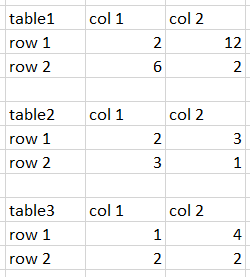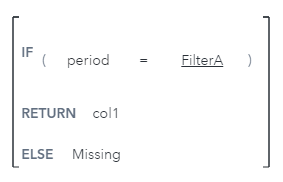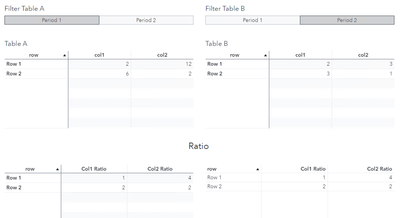## SAS VA; difference/ratio between two tables that are the same, but using different filters

Hi all,

I have two tables that are exactly the same. Same data, row, columns etc.

I have given them a filter each; both for "period". I want to be able to see Period 1 and Period 2 at the same time.

Is there now a way for me to add a third table, which displays the ratio between the cells in the two tables, when I have filtered for different periods in the two tables?

For example.Best regards,

GustavRenato_sas
SAS Employee

## Re: SAS VA; difference/ratio between two tables that are the same, but using different filters

Hi @Gust ,

I've assigned parameters to the filters (button bars in my example) to capture the period selection on each table. Parameters were called FilterA and FilterB.

Then I've created 4 calculations for FilterA_col1, FilterA_col2, FilterB_col1, and FilterB_col2. Their expressions are very similar, Here is the expression for FilterA_col1:Then I calculated the ratio between FilterA_col1 and FilterB_col1 and called it "Col1 Ratio", and then did the same for col2. This is the expression for "Col1 Ratio":This is the result:I hope this is what you wanted.

Discussion stats